Sunil Ray — Updated On May 31st, 2020

This article was originally published on November 18, 2015, and updated on April 30, 2018.

## Introduction

One of the most interesting and challenging things about data science hackathons is getting a high score on both public and private leaderboards. I have closely monitored the series of data science hackathons and found an interesting trend. This trend is based on participant rankings on the public and private leaderboards.

One thing that stood out was that participants who rank higher on the public leaderboard lose their position after their ranks gets validated on the private leaderboard. Some even failed to secure rank in the top 20s on the private leaderboard (image below).

Eventually, I discovered the phenomenon which brings such ripples on the leaderboard.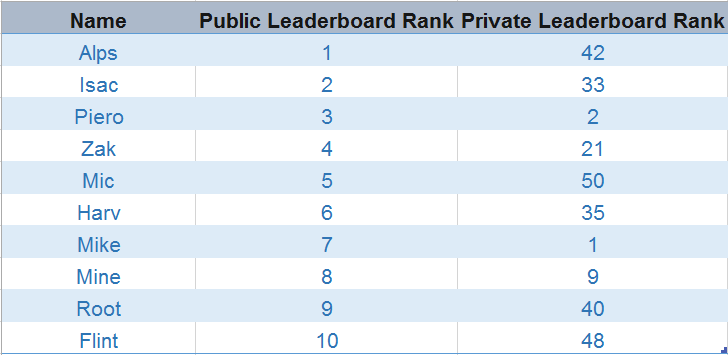Take a guess! What could be the possible reason for high variation in these ranks? In other words, why does their model lose stability when evaluated on the private leaderboard?

In this article, we will look at possible reasons for this. We will also look at the concept of cross validation and a few common methods to perform it.

Note: This article is meant for every aspiring data scientist keen to improve his/her performance in data science competitions. Each technique is followed by code snippets from both R and Python.

1. Why do models lose stability?
2. What is cross validation?
3. A few common methods used for cross validation
• The Validation set Approach
• Leave out one cross validation (LOOCV)
• k-fold cross validation
• Stratified k-fold cross validation
• Cross validation for time series
• Custom cross validation techniques
4. How to measure the model’s bias-variance?

## Why do models lose stability?

Let’s understand this using the below snapshot illustrating the fit of various models: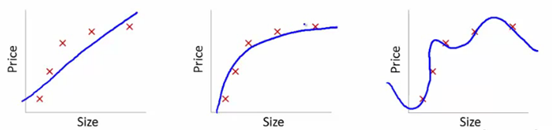Here, we are trying to find the relationship between size and price. To achieve this, we have taken the following steps:

1. We’ve established the relationship using a linear equation for which the plots have been shown. The first plot has a high error from training data points. Therefore, this will not perform well on either public or the private leaderboard. This is an example of “Underfitting”. In this case, our model fails to capture the underlying trend of the data
2. In the second plot, we just found the right relationship between price and size, i.e., low training error and generalization of the relationship
3. In the third plot, we found a relationship which has almost zero training error. This is because the relationship is developed by considering each deviation in the data point (including noise), i.e., the model is too sensitive and captures random patterns which are present only in the current dataset. This is an example of “Overfitting”. In this relationship, there could be a high deviation between the public and private leaderboards

A common practice in data science competitions is to iterate over various models to find a better performing model. However, it becomes difficult to distinguish whether this improvement in score is coming because we are capturing the relationship better, or we are just over-fitting the data. To find the right answer for this question, we use validation techniques. This method helps us in achieving more generalized relationships.

## What is Cross Validation?

Cross Validation is a technique which involves reserving a particular sample of a dataset on which you do not train the model. Later, you test your model on this sample before finalizing it.

Here are the steps involved in cross validation:

1. You reserve a sample data set
2. Train the model using the remaining part of the dataset
3. Use the reserve sample of the test (validation) set. This will help you in gauging the effectiveness of your model’s performance. If your model delivers a positive result on validation data, go ahead with the current model. It rocks!

## A few common methods used for Cross Validation

There are various methods available for performing cross validation. I’ve discussed a few of them in this section.

### The validation set approach

In this approach, we reserve 50% of the dataset for validation and the remaining 50% for model training. However, a major disadvantage of this approach is that since we are training a model on only 50% of the dataset, there is a huge possibility that we might miss out on some interesting information about the data which will lead to a higher bias.

Python Code:

`train, validation = train_test_split(data, test_size=0.50, random_state = 5)`

R Code:

```set.seed(101) # Set Seed so that same sample can be reproduced in future also

# Now Selecting 50% of data as sample from total 'n' rows of the data
sample <- sample.int(n = nrow(data), size = floor(.50*nrow(data)), replace = F)
train <- data[sample, ]
test  <- data[-sample, ]```

### Leave one out cross validation (LOOCV)

In this approach, we reserve only one data point from the available dataset, and train the model on the rest of the data. This process iterates for each data point. This also has its own advantages and disadvantages. Let’s look at them:

• We make use of all data points, hence the bias will be low
• We repeat the cross validation process n times (where n is number of data points) which results in a higher execution time
• This approach leads to higher variation in testing model effectiveness because we test against one data point. So, our estimation gets highly influenced by the data point. If the data point turns out to be an outlier, it can lead to a higher variation

Python Code:

R Code:

```score = list()

LOOCV_function = function(x,label){
for(i in 1:nrow(x)){
training = x[-i,]
model = #... train model on training
validation = x[i,]
pred = predict(model, validation[,setdiff(names(validation),label)])
score[[i]] = rmse(pred, validation[[label]]) # score/error of ith fold
}
return(unlist(score)) # returns a vector
}```

LOOCV leaves one data point out. Similarly, you could leave p training examples out to have validation set of size p for each iteration. This is called LPOCV (Leave P Out Cross Validation)

### k-fold cross validation

From the above two validation methods, we’ve learnt:

1. We should train the model on a large portion of the dataset. Otherwise we’ll fail to read and recognise the underlying trend in the data. This will eventually result in a higher bias
2. We also need a good ratio of testing data points. As we have seen above, less amount of data points can lead to a variance error while testing the effectiveness of the model
3. We should iterate on the training and testing process multiple times. We should change the train and test dataset distribution. This helps in validating the model effectiveness properly

Do we have a method which takes care of all these 3 requirements?

Yes! That method is known as “k-fold cross validation”. It’s easy to follow and implement. Below are the steps for it:

1. Randomly split your entire dataset into k”folds”
2. For each k-fold in your dataset, build your model on k – 1 folds of the dataset. Then, test the model to check the effectiveness for kth fold
3. Record the error you see on each of the predictions
4. Repeat this until each of the k-folds has served as the test set
5. The average of your k recorded errors is called the cross-validation error and will serve as your performance metric for the model

Below is the visualization of a k-fold validation when k=10.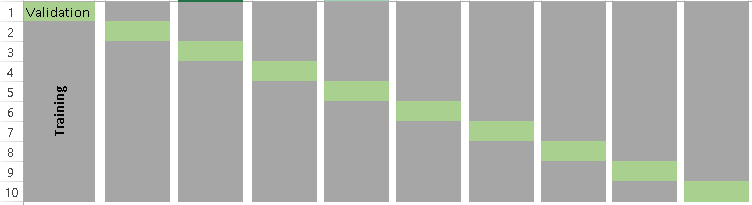Now, one of most commonly asked questions is, “How to choose the right value of k?”.

Always remember, a lower value of k is more biased, and hence undesirable. On the other hand, a higher value of K is less biased, but can suffer from large variability. It is important to know that a smaller value of k always takes us towards validation set approach, whereas a higher value of k leads to LOOCV approach.

Precisely, LOOCV is equivalent to n-fold cross validation where n is the number of training examples.

Python Code:

```from sklearn.model_selection import KFold
kf = RepeatedKFold(n_splits=5, n_repeats=10, random_state=None)

for train_index, test_index in kf.split(X):
print("Train:", train_index, "Validation:",test_index)
X_train, X_test = X[train_index], X[test_index]
y_train, y_test = y[train_index], y[test_index]```

R code:

```library(caret)
data(iris)

# Define train control for k fold cross validation
train_control <- trainControl(method="cv", number=10)
# Fit Naive Bayes Model
model <- train(Species~., data=iris, trControl=train_control, method="nb")
# Summarise Results
print(model)```

### 4. Stratified k-fold cross validation

Stratification is the process of rearranging the data so as to ensure that each fold is a good representative of the whole. For example, in a binary classification problem where each class comprises of 50% of the data, it is best to arrange the data such that in every fold, each class comprises of about half the instances.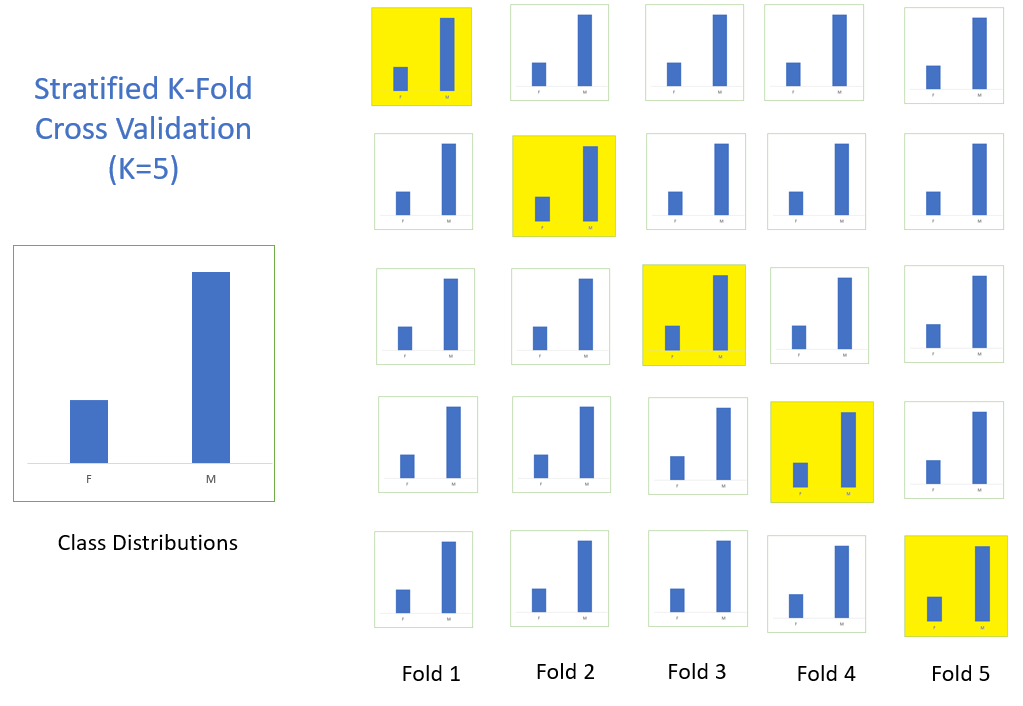It is generally a better approach when dealing with both bias and variance. A randomly selected fold might not adequately represent the minor class, particularly in cases where there is a huge class imbalance.

Python code snippet for stratified k-fold cross validation:

```from sklearn.model_selection import StratifiedKFold
skf = StratifiedKFold(n_splits=5, random_state=None)
# X is the feature set and y is the target
for train_index, test_index in skf.split(X,y):
print("Train:", train_index, "Validation:", val_index)
X_train, X_test = X[train_index], X[val_index]
y_train, y_test = y[train_index], y[val_index]

```

R Code:

```library(caret)
# Folds are created on the basis of target variable
folds <- createFolds(factor(data\$target), k = 10, list = FALSE)```

Having said that, if the train set does not adequately represent the entire population, then using a stratified k-fold might not be the best idea. In such cases, one should use a simple k-fold cross validation with repetition.

In repeated cross-validation, the cross-validation procedure is repeated n times, yielding n random partitions of the original sample. The n results are again averaged (or otherwise combined) to produce a single estimation.

Python code for repeated k-fold cross validation:

```from sklearn.model_selection import RepeatedKFold
rkf = RepeatedKFold(n_splits=5, n_repeats=10, random_state=None)
# X is the feature set and y is the target
for train_index, test_index in rkf.split(X):
print("Train:", train_index, "Validation:", val_index)
X_train, X_test = X[train_index], X[val_index]
y_train, y_test = y[train_index], y[val_index]
```

When dealing with real datasets, there are often cases where the test and train sets are very different. As a result, the internal cross-validation techniques might give scores that are not even in the ballpark of the test score. In such cases, adversarial validation offers an interesting solution.

The general idea is to check the degree of similarity between training and tests in terms of feature distribution. If It does not seem to be the case, we can suspect they are quite different. This intuition can be quantified by combining train and test sets, assigning 0/1 labels (0 – train, 1-test) and evaluating a binary classification task.

Let us understand, how this can be accomplished in the below steps:

1. Remove the target variable from the train set
`train.drop(['target'], axis = 1, inplace = True)`
1. Create a new target variable which is 1 for each row in the train set, and 0 for each row in the test set
```train['is_train'] = 1
test['is_train'] = 0```
1. Combine the train and test datasets
`df = pd.concat([train, test], axis = 0)`
1. Using the above newly created target variable, fit a classification model and predict probabilities for each row to be in the test set
```y = df['is_train']; df.drop('is_train', axis = 1, inplace = True)
# Xgboost parameters
xgb_params = {'learning_rate': 0.05,
'max_depth': 4,
'subsample': 0.9,
'colsample_bytree': 0.9,
'objective': 'binary:logistic',
'silent': 1,
'n_estimators':100,
'gamma':1,
'min_child_weight':4}
clf = xgb.XGBClassifier(**xgb_params, seed = 10)```
1. Sort the train set using the calculated probabilities in step 4 and take top n% samples/rows as the validation set (n% is the fraction of the train set you want to keep in the validation set)
```probs = clf.predict_proba(x1)[:,1]
new_df = pd.DataFrame({'id':train.id, 'probs':probs})
new_df = new_df.sort_values(by = 'probs', ascending=False) # 30% validation set
val_set_ids = new_df.iloc[1:np.int(new_df.shape*0.3),1]```

val_set_ids will get you the ids from the train set that would constitute the validation set which is most similar to the test set. This will make your validation strategy more robust for cases where the train and test sets are highly dissimilar.

However, you must be careful while using this type of validation technique. Once the distribution of the test set changes, the validation set might no longer be a good subset to evaluate your model on.

### 6. Cross Validation for time series

Splitting a time-series dataset randomly does not work because the time section of your data will be messed up. For a time series forecasting problem, we perform cross validation in the following manner.

1. Folds for time series cross valdiation are created in a forward chaining fashion
2. Suppose we have a time series for yearly consumer demand for a product during a period of n years. The folds would be created like:
```fold 1: training , test 
fold 2: training [1 2], test 
fold 3: training [1 2 3], test 
fold 4: training [1 2 3 4], test 
fold 5: training [1 2 3 4 5], test 
.
.
.
fold n: training [1 2 3 ….. n-1], test [n]```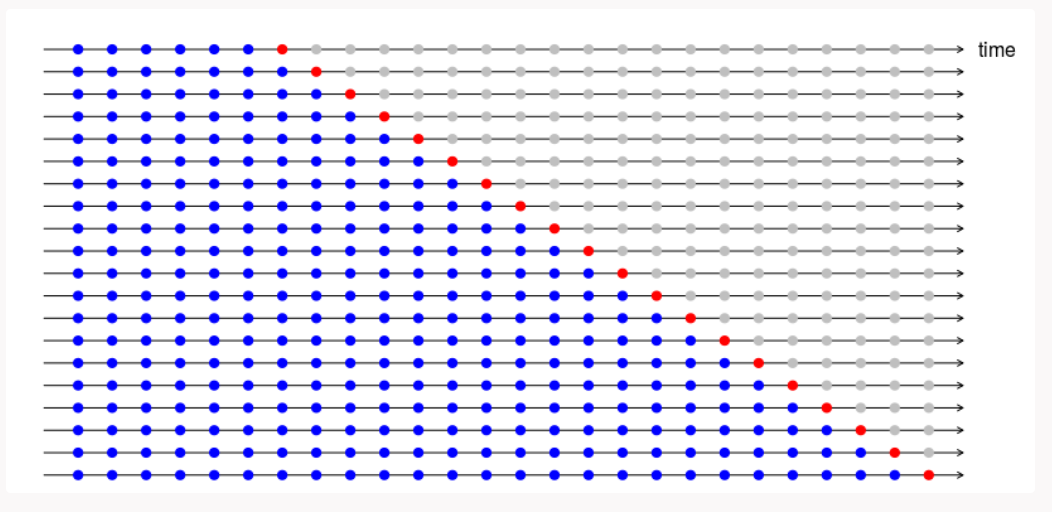We progressively select a new train and test set. We start with a train set which has a minimum number of observations needed for fitting the model. Progressively, we change our train and test sets with each fold. In most cases, 1 step forecasts might not be very important. In such instances, the forecast origin can be shifted to allow for multi-step errors to be used. For example, in a regression problem, the following code could be used for performing cross validation.

Python Code:

```from sklearn.model_selection import TimeSeriesSplit
X = np.array([[1, 2], [3, 4], [1, 2], [3, 4]])
y = np.array([1, 2, 3, 4])
tscv = TimeSeriesSplit(n_splits=3)
```
```for train_index, test_index in tscv.split(X):
print("Train:", train_index, "Validation:", val_index)
X_train, X_test = X[train_index], X[val_index]
y_train, y_test = y[train_index], y[val_index]

TRAIN:  TEST: 
TRAIN: [0 1] TEST: 
TRAIN: [0 1 2] TEST: ```

R Code:

```library(fpp)
library(forecast)
e <- tsCV(ts, Arima(x, order=c(2,0,0), h=1) #CV for arima model
sqrt(mean(e^2, na.rm=TRUE)) # RMSE```

h = 1 implies that we are taking the error only for 1 step ahead forecasts.

(h =4) 4-step ahead error is depicted in the below diagram. This could be used if you want to evaluate your model for multi-step forecast.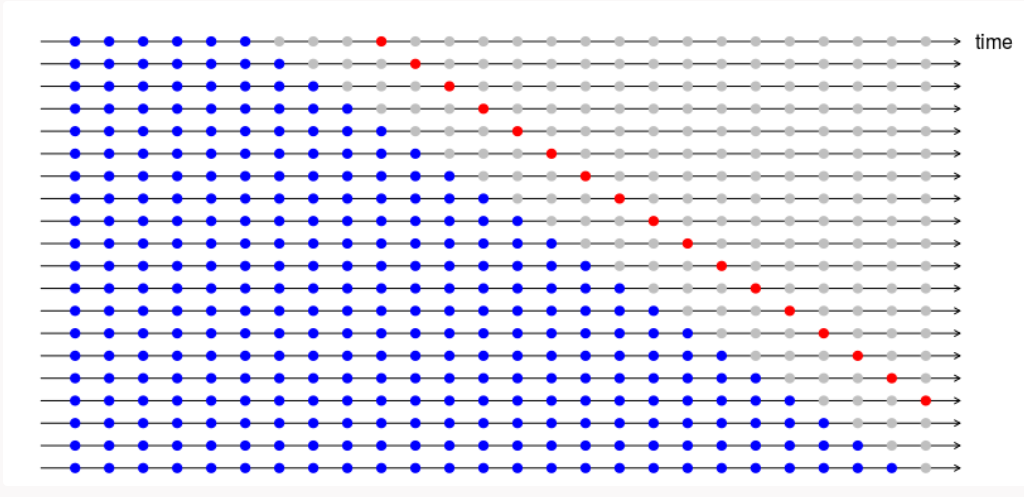### 7. Custom Cross Validation Techniques

Unfortunately, there is no single method that works best for all kinds of problem statements. Often, a custom cross validation technique based on a feature, or combination of features, could be created if that gives the user stable cross validation scores while making submissions in hackathons.

For example, in the recently finished contest ‘Lord of the Machines‘ by Analytics Vidhya, the most stable validation technique used by the top finishers was using the campaign id variable.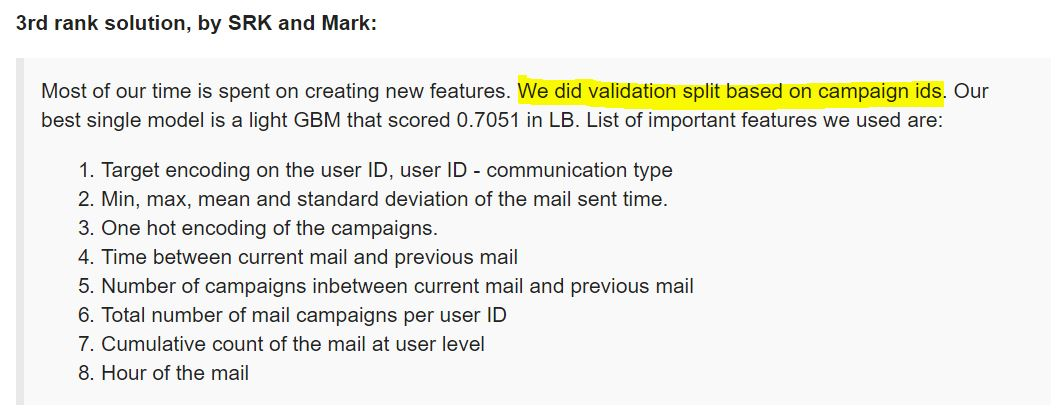Please have a look at the problem statement and a few approaches discussed by the participants at this thread.

## How to measure the model’s bias-variance?

After k-fold cross validation, we’ll get k different model estimation errors (e1, e2 …..ek). In an ideal scenario, these error values should sum up to zero. To return the model’s bias, we take the average of all the errors. Lower the average value, better the model.

Similarly for calculating the model variance, we take standard deviation of all the errors. A low value of standard deviation suggests our model does not vary a lot with different subsets of training data.

We should focus on achieving a balance between bias and variance. This can be done by reducing the variance and controlling bias to an extent. It’ll result in a better predictive model. This trade-off usually leads to building less complex predictive models as well. For understanding bias-variance trade-off in more depth, please refer to section 9 of this article.

## End Notes

In this article, we discussed about overfitting and methods like cross-validation to avoid overfitting. We also looked at different cross-validation methods like validation set approach, LOOCV, k-fold cross validation, stratified k-fold and so on, followed by each approach’s implementation in Python and R performed on the Iris dataset.###### Sunil Ray

I am a Business Analytics and Intelligence professional with deep experience in the Indian Insurance industry. I have worked for various multi-national Insurance companies in last 7 years.

## 31 thoughts on "Improve Your Model Performance using Cross Validation (in Python and R)"###### Muthupandi says:November 19, 2015 at 6:43 am
Thank you for good explained article. Am just starting to explore the Analytics for past 6 months, Cross validation is the one i was looking to get my hands on and got ur article. One question is what is the Error Function we need to use? Reply###### Akash says:November 19, 2015 at 6:01 pm
Thanks sunil for the article. Is this the general way of writing the code in R and python for cross-validation? or are there other ways? Thanks, Reply###### Neehar says:November 20, 2015 at 1:42 am
Hi Sunil , Thank you . Great article. Reply###### Neehar says:November 20, 2015 at 1:49 am
I have a question. if we are creating 10 fold cross validation, we are training a model on 10 different datasets. It means we get 10 instances of model trained on 10 different datasets. At the time of final prediction do we need to predict our data on these 10 instances of models ? or take the skeleton of the model (same options we have used in CV) and train it on whole dataset and predict ? Can anyone please clarify ? Reply###### Ram says:November 22, 2015 at 4:57 pm
Neehar, Good question. Perhaps this will help you: If you are working in R, you can use the caret library to do the same processing with fewer lines of code. Note: The train function tries several models, and selects the best model. ### Load the library library(caret) # Load the iris dataset data(iris) # Define training control: 5 fold cross-validation. If you want to perform 10 fold cv, set number=10, train_control <- trainControl(method="cv", number=5) # Train the model using randomForest (rf) model <- train(Sepal.Length~., data=iris, trControl=train_control, method="rf") ##The printed summary shows the sample sizes used, the best model selected and other information. print(model) # Make predictions predictions <- predict(model, iris[,-1]) # Summarize results result <- data.frame(Actual=iris[,1],Predicted=predictions) result\$Difference <- abs(result\$Actual - result\$Predicted) summary(result\$Difference) ## The End Reply###### venugopal says:November 28, 2015 at 5:02 pm###### Rajesh says:December 29, 2015 at 8:48 am
Hi, Nice article explaining k-fold cross validation. Just thought of adding the mae function from hydroGOF package library(hydroGOF) sim <- result\$Predicted obs <- result\$Actual mae(sim,obs) Output : 0.3157678 Reply###### selmi says:January 15, 2016 at 10:17 am
thanks for your good article , i have a question if you can explaine more please in fact : i have tested the tow appeoch of cross validation by using your script in the first hand and by using caret package as you mentioned in your comment : why in the caret package the sample sizes is always around 120,121... it is possible to get for example sample sizes 140 or 90 .. No pre-processing Resampling: Cross-Validated (5 fold) Summary of sample sizes: 120, 121, 119, 120, 120 Resampling results across tuning parameters: any clarification please about functioning of this method. thanks in advance Reply###### Ram says:January 15, 2016 at 4:05 pm
@Semi, :'Why in the caret package the sample sizes is always around 120,121…" A good question. Answer: The 'sample size used by caret' depends on the resampling parameter provided in trainControl. It seems that you used the value 5.. Try it again with a value 10. You will see a different sample size selected. tc <- trainControl("cv", number=10) model <- train(Sepal.Length~., data=iris, trControl=train_control, method="rf") Hope this helps. Reply###### selmi says:January 15, 2016 at 4:49 pm
thanks for the reply but can you expaine to me "the resampling paramet" when we use resamling is not a bootstrap ? thanks Reply###### Ram says:January 16, 2016 at 4:10 pm
The resampling method ('bootstrap' or 'no bootstrap') depends on the parameter specified in trainControl. You can try the following, and see the results: For the benefit of others, please describe what you see. tc.cv <- trainControl("cv", number=10) model1 <- train(Sepal.Length~., data=iris, trControl=tc.cv, method="rf") print (model1) tc.boot <- trainControl("boot", number=10) model2 <- train(Sepal.Length~., data=iris, trControl=tc.boot, method="rf") print (model2) Reply###### selmi says:January 18, 2016 at 9:54 am
@Ram thanks for your clarification , when i have compare two methos (cv and boot) , i remark that for model 1 (with cv,n=5 ) summary of sample sizes are: 120, 121, 120, 120, 119 and for the model 2 (with boot,n=5) Summary of sample sizes: 150, 150, 150, 150, 150 so can you telle me how can i use cv or boot method ? should i compare RMSE for each method and take the smaller value? waiting your reply thanks in advance . Reply###### Ram says:January 18, 2016 at 4:10 pm
@Selmi, You might want to read the article again. Sunil has explained it. (How to measure the model’s bias-variance?) Reply###### Ashish Yelkar says:October 05, 2016 at 7:34 am###### Tatsiana says:April 14, 2017 at 7:47 am###### Sameer says:August 06, 2017 at 2:00 am
Thanks Sunil, Very nice article.. R users can switch to using caret as library for cross validation. It's has a standardized wrapper for all the models and cross validation.. Traincontrl is the parameter name.. Reply###### Priyanshu says:March 08, 2018 at 12:14 pm
what if cross validation score becomes zero after the models are trained? Which conclusion we will come to? Model is appropriate or failed ?? Reply###### Arpit says:March 29, 2018 at 8:42 pm
This is completely irrelevant topic. Model performance will never improve. Model Accuracy can be rightly captured by K fold validation. Final model is always on all data. So irrespective of using k fold, non k fold, final model will always be same. Reply###### Aishwarya Singh says:April 04, 2018 at 1:42 pm
Hi Arpit, It is correct that the final model will be on the whole dataset but using cross validation, we can tune our parameters effectively to improve the overall model performance. Reply###### Ankit Choudhary says:April 04, 2018 at 7:37 pm###### Mario says:May 14, 2018 at 8:34 pm
Great Article! The following part of the code placed on time series has a mistake: from sklearn.model_selection import TimeSeriesSplit from sklearn.model_selection import TimeSeriesSplit X = np.array([[1, 2], [3, 4], [1, 2], [3, 4]]) y = np.array([1, 2, 3, 4]) tscv = TimeSeriesSplit(n_splits=3) for train_index, test_index in tscv.split(X): print("Train:", train_index, "Validation:", val_index) X_train, X_test = X[train_index], X[val_index] y_train, y_test = y[train_index], y[val_index] -> val_index is not deffined it should be test_index I think :) X = np.array([[1, 2], [3, 4], [1, 2], [3, 4]]) y = np.array([1, 2, 3, 4]) tscv = TimeSeriesSplit(n_splits=3) for train_index, test_index in tscv.split(X): print("Train:", train_index, "Validation:", test_index) X_train, X_test = X[train_index], X[test_index] y_train, y_test = y[train_index], y[test_index] Reply###### Faizan Shaikh says:May 17, 2018 at 3:56 pm
Thanks for the suggestion! We have updated the code. Reply###### Vignesh says:June 05, 2018 at 6:49 am
Very informative Blog... Thanks Sunil!! Reply###### Ankit Choudhary says:June 05, 2018 at 5:41 pm
Hi Priyanshu, What kind of problem are you trying to solve Regression/Classification? What is the evaluation metric? Reply###### Shanal Agrawal says:June 06, 2018 at 10:59 am
Thanks for a great article to understand the use of cross-validation techniques for a good fit of the model. Reply###### DEB says:July 07, 2018 at 10:46 am
Can anyone please answer what kind of split mechanism is ideal for a very imbalanced dataset in a classification problem ? Is it K-Fold, StratifiedKfold or ShuffleSplit ? I have the total dataset count of 3338. Out of which Class A count is 525, Class B counts 2134 and Class C counts 679. So, the dataset is having almost 70% of a particular class B and rest is divided amongst class A & C. Please suggest. FYIP, I am using python. Thanks Reply###### Pulkit Sharma says:July 10, 2018 at 2:26 pm
Hi DEB, You can use StratifiedkFold as it will keep equal ratio of each class in every split making each split silimar. Reply###### Gemma says:December 19, 2018 at 4:40 pm
Thanks @Sunil for this great article! Very useful :) About this code that @RAM wrote back in November 2015, my question is: if I want to make sure my final model is not overfitted (even though RF seems to prevent overfitting), do I compare these results (code below) from the predictions, against the OOB error? I understand the former is the error in the CV trained datasets, and the latter is the error in the CV test datasets. Is this correct? Many thanks! # Make predictions predictions <- predict(model, iris[,-1]) # Summarize results result <- data.frame(Actual=iris[,1],Predicted=predictions) result\$Difference <- abs(result\$Actual – result\$Predicted) summary(result\$Difference) Reply###### Pulkit Sharma says:December 19, 2018 at 5:03 pm
Hi Gemma, If the validation accuracy is in sync with the training accuracy, you can say that the model is not overfitting. If the training accuracy is increasing while the validation accuracy starts to decrease, then the model is overfitting. Reply###### Manan says:February 05, 2023 at 12:06 am
A great article explains the practical usage of cross-validation. Another article that I liked a lot can be found at Reply###### Subash says:June 24, 2023 at 7:19 am
Very good explanation of the need of Cross-validation in ML Reply